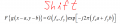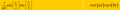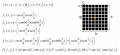# creating shapes using fourier transform

#### yef smith

Joined Aug 2, 2020
568
Hello, there is an example where we need to do fourier transform to a shape shown bellow.
my log is to take the small black square and shift it in the locations as shown bellow.
So we need the double sinc function and to do a convolution with delta cones to create it.

but in the solution they did two double sinc functions,why is that?
what is the logic intuition of the shown bellow?
Thanks.Solution: